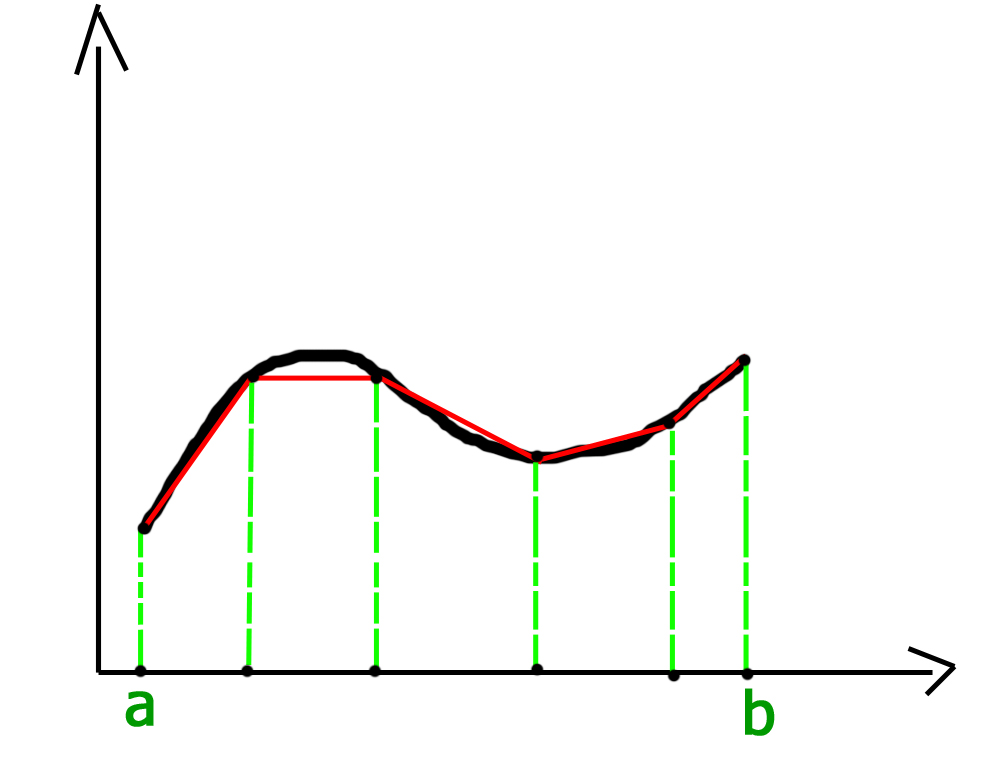# Trapezoidal Rule for Approximate Value of Definite Integral

In the field of numerical analysis, Trapezoidal rule is used to find the approximation of a definite integral. The basic idea in Trapezoidal rule is to assume the region under the graph of the given function to be a trapezoid and calculate its area.
It follows that:For more accurate results the domain of the graph is divided into n segments of equal size as shown below:Grid spacing or segment size h = (b-a) / n.
Therefore, approximate value of the integral can be given by:## C++

 // C++ program to implement Trapezoidal rule  #include     // A sample function whose definite integral's  // approximate value is computed using Trapezoidal  // rule  float y(float x)  {      // Declaring the function f(x) = 1/(1+x*x)      return 1/(1+x*x);  }     // Function to evaluate the value of integral  float trapezoidal(float a, float b, float n)  {      // Grid spacing      float h = (b-a)/n;         // Computing sum of first and last terms      // in above formula      float s = y(a)+y(b);         // Adding middle terms in above formula      for (int i = 1; i < n; i++)          s += 2*y(a+i*h);         // h/2 indicates (b-a)/2n. Multiplying h/2      // with s.      return (h/2)*s;  }     // Driver program to test above function  int main()  {      // Range of definite integral      float x0 = 0;      float xn = 1;         // Number of grids. Higher value means      // more accuracy      int n = 6;         printf("Value of integral is %6.4f\n",                    trapezoidal(x0, xn, n));      return 0;  }

## Java

 // Java program to implement Trapezoidal rule     class GFG  {      // A sample function whose definite      // integral's approximate value        // is computed using Trapezoidal      // rule      static float y(float x)      {          // Declaring the function           // f(x) = 1/(1+x*x)          return 1 / (1 + x * x);      }             // Function to evaluate the value of integral      static float trapezoidal(float a, float b, float n)      {          // Grid spacing          float h = (b - a) / n;                 // Computing sum of first and last terms          // in above formula          float s = y(a) + y(b);                 // Adding middle terms in above formula          for (int i = 1; i < n; i++)              s += 2 * y( a + i * h);                 // h/2 indicates (b-a)/2n. Multiplying h/2          // with s.          return (h / 2) * s;      }             // Driver code      public static void main (String[] args)      {          // Range of definite integral          float x0 = 0;          float xn = 1;                 // Number of grids. Higher           // value means more accuracy          int n = 6;                 System.out.println("Value of integral is "+                             Math.round(trapezoidal(x0, xn, n)                              * 10000.0) / 10000.0);      }  }     // This code is contributed by Anant Agarwal.

## Python3

 # Python3 code to implement Trapezoidal rule     # A sample function whose definite   # integral's approximate value is   # computed using Trapezoidal rule  def y( x ):             # Declaring the function       # f(x) = 1/(1+x*x)      return (1 / (1 + x * x))         # Function to evaluate the value of integral  def trapezoidal (a, b, n):             # Grid spacing      h = (b - a) / n             # Computing sum of first and last terms      # in above formula      s = (y(a) + y(b))         # Adding middle terms in above formula      i = 1     while i < n:          s += 2 * y(a + i * h)          i += 1                # h/2 indicates (b-a)/2n.       # Multiplying h/2 with s.      return ((h / 2) * s)     # Driver code to test above function  # Range of definite integral  x0 = 0 xn = 1    # Number of grids. Higher value means  # more accuracy  n = 6 print ("Value of integral is ",       "%.4f"%trapezoidal(x0, xn, n))        # This code is contributed by "Sharad_Bhardwaj".

## C#

 // C# program to implement Trapezoidal  // rule.  using System;     class GFG {             // A sample function whose definite      // integral's approximate value       // is computed using Trapezoidal      // rule      static float y(float x)      {                     // Declaring the function           // f(x) = 1/(1+x*x)          return 1 / (1 + x * x);      }             // Function to evaluate the value       // of integral      static float trapezoidal(float a,                          float b, float n)      {                     // Grid spacing          float h = (b - a) / n;                 // Computing sum of first and           // last terms in above formula          float s = y(a) + y(b);                 // Adding middle terms in above          // formula          for (int i = 1; i < n; i++)              s += 2 * y( a + i * h);                 // h/2 indicates (b-a)/2n.           // Multiplying h/2 with s.          return (h / 2) * s;      }             // Driver code      public static void Main ()      {                     // Range of definite integral          float x0 = 0;          float xn = 1;                 // Number of grids. Higher           // value means more accuracy          int n = 6;                 Console.Write("Value of integral is "           + Math.Round(trapezoidal(x0, xn, n)                           * 10000.0) / 10000.0);      }  }     // This code is contributed by nitin mittal.

## PHP

 

## Javascript

 

Output:

Value of integral is 0.7842

If you like GeeksforGeeks and would like to contribute, you can also write an article using write.geeksforgeeks.org or mail your article to review-team@geeksforgeeks.org. See your article appearing on the GeeksforGeeks main page and help other Geeks.